# Test: Semiconductor Physics

## 20 Questions MCQ Test GATE Electrical Engineering (EE) 2023 Mock Test Series | Test: Semiconductor Physics

Description
Attempt Test: Semiconductor Physics | 20 questions in 60 minutes | Mock test for Electrical Engineering (EE) preparation | Free important questions MCQ to study GATE Electrical Engineering (EE) 2023 Mock Test Series for Electrical Engineering (EE) Exam | Download free PDF with solutions
QUESTION: 1

### In germanium semiconductor material at T = 400 K the intrinsic concentration is

Solution: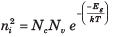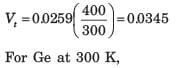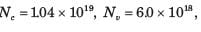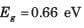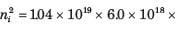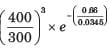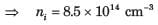QUESTION: 2

### The intrinsic carrier concentration in silicon is to be no greater than ni  = 1x1012 cm-3 . The maximum temperature allowed for the silicon is (Eg = 1.12 eV)

Solution: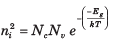(1012)2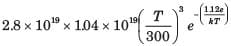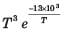= 9.28 x 10-8,  By trial T = 385 K

QUESTION: 3

### Two semiconductor material have exactly the same properties except that material A has a bandgap of 1.0eV and material B has a bandgap energy of 1.2 eV. The ratio of intrinsic concentration of material A to that of material B is

Solution: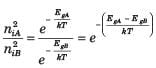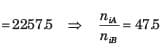QUESTION: 4

In silicon at T = 300 K the thermal-equilibrium concentration of electron is n0 = 5 x 104 cm-3 The hole concentration is

Solution: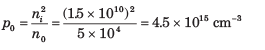QUESTION: 5

In silicon at T = 300 K if the Fermi energy is 0.22 eV above the valence band energy, the value of p0 is

Solution: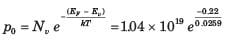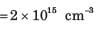QUESTION: 6

The thermal-equilibrium concentration of hole p0 in silicon at T =  300 K is 1015  cm-3. The value of n0 is

Solution: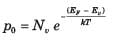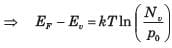At 300 K, Nv = 1.0 x 1019 cm-3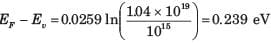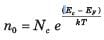At 300 K, Nc = 2.8 x 1019 cm-3
Ec - EF = 1.12 - 0.239 = 0.881 eV
n = 4.4 x 104 cm-3

QUESTION: 7

In germanium semiconductor at T = 300 K, the acceptor concentrations is Na = 1013 cm-3 and donor concentration is Nd = 0. The thermal equilibrium concentration p0 is

Solution: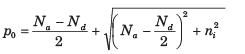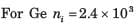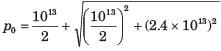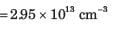QUESTION: 8

A thin film resistor is to be made from a GaAs film doped n - type. The resistor is to have a value of 2 kΩ. The resistor length is to be 200 μm and area is to be 10-6 cm2. The doping efficiency is known to be 90%. The mobility of electrons is 8000 cm2 / V - s . The doping needed is

Solution: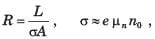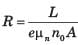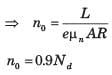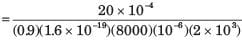QUESTION: 9

A silicon sample doped n - type at 1018 cm-3  have a resistance of 10 Ω . The sample has an area of 10-6 cm2 and a length of 10 μm. The doping efficiency of the sample is (μn =  800 cm2/V - s)

Solution: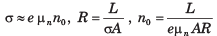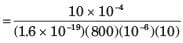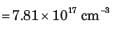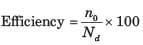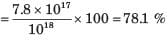QUESTION: 10

Six volts is applied across a 2 cm long semiconductor bar. The average drift velocity is 10cm/s. The electron mobility is

Solution: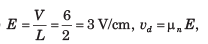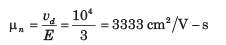QUESTION: 11

For a particular semiconductor material following parameters are observed: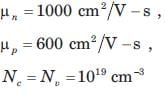These parameters are independent of temperature. The measured conductivity of the intrinsic material is σ = 10-6 (Ω - cm)-1 at T = 300 K. The conductivity at T = 500 K is

Solution: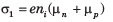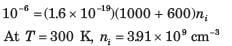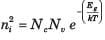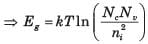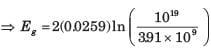= 1.122 eV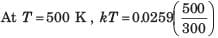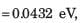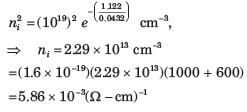QUESTION: 12

An n - type silicon sample has a resistivity of 5 Ω - cm at T = 300 K. The mobility is μn = 1350 cm2 /  V - s. The donor impurity concentration is

Solution: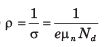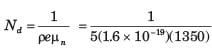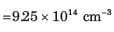QUESTION: 13

In a silicon sample the electron concentration drops linearly from 1018 cm-3  to 1016 cm-3 over a length of 2.0 μm. The current density due to the electron diffusion current is D= 35cm2/s

Solution: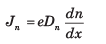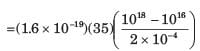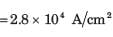QUESTION: 14

In a GaAs sample the electrons are moving under an electric field of 5 kV cm and the carrier concentration is uniform at 1016 cm-3. The electron velocity is the saturated velocity of 107 cm/s. The drift current density is

Solution:

J = evn = (1.6 x 10-19)(107)(1016) = 1.6 x 104 A/cm2

QUESTION: 15

For a sample of GaAs scattering time is τsc =  10-13s and electron’s effective mass is me* =  0.067 mo. If an electric field of 1 kV cm is applied, the drift velocity produced is

Solution: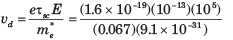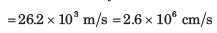QUESTION: 16

A gallium arsenide semiconductor at T = 300 K is doped with impurity concentration Nd = 1016 cm-3 The mobility μn is 7500 cm2/ V - s  . For an applied field of 10 V/cm the drift current density is

Solution: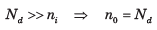J = eμn n0E = (1.6 x 10-19)(7500)(1016)(10) = 120 A/cm2

QUESTION: 17

In a particular semiconductor the donor impurity concentration is Nd = 1014 cm-3. Assume the following parameters,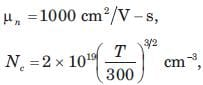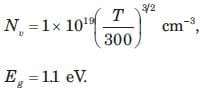An electric field of E = 10 V cm is applied. The electric current density at 300 K is

Solution: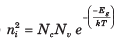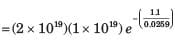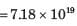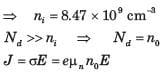=(1.6 x 10-19)(1000)(1014)(100) = 1.6 A/cm2

QUESTION: 18

A semiconductor has following parameter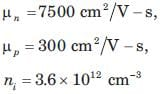Q. When conductivity is minimum, the hole concentration is

Solution: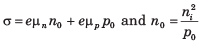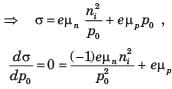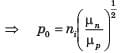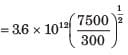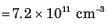QUESTION: 19

A semiconductor has following parameter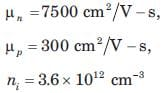Q. The minimum conductivity is

Solution: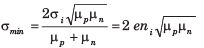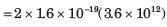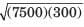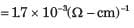QUESTION: 20

A particular intrinsic semiconductor has a resistivity of 50 (Ω - cm) at T = 300 K and 5(Ω - cm) at T = 330 K. If change in mobility with temperature is neglected, the bandgap energy of the semiconductor is

Solution: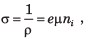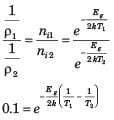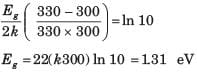Use Code STAYHOME200 and get INR 200 additional OFF Use Coupon Code